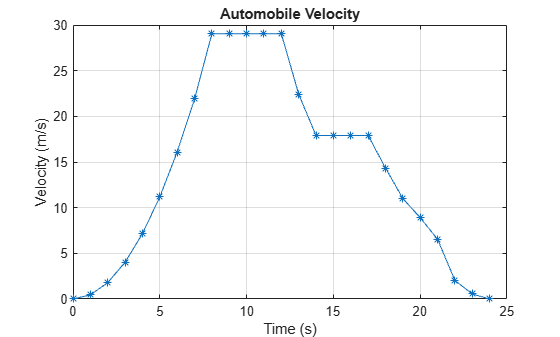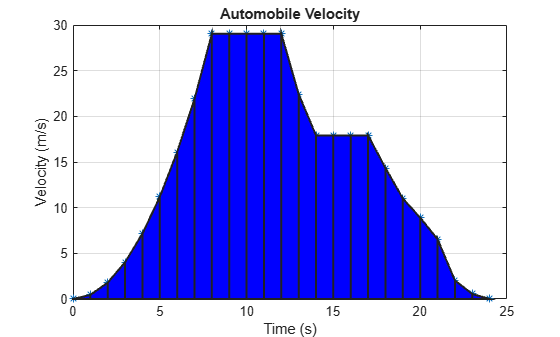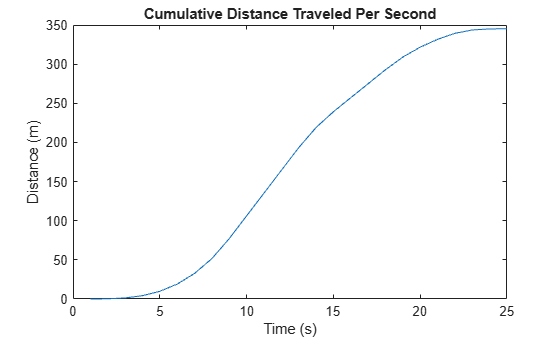Main Content

# Integration of Numeric Data

This example shows how to integrate a set of discrete velocity data numerically to approximate the distance traveled. The `integral` family only accepts function handles as inputs, so those functions cannot be used with discrete data sets. Use `trapz` or `cumtrapz` when a functional expression is not available for integration.

### View the Velocity Data

Consider the following velocity data and corresponding time data.

```vel = [0 .45 1.79 4.02 7.15 11.18 16.09 21.90 29.05 29.05 ... 29.05 29.05 29.05 22.42 17.9 17.9 17.9 17.9 14.34 11.01 ... 8.9 6.54 2.03 0.55 0]; time = 0:24;```

This data represents the velocity of an automobile (in m/s) taken at 1 s intervals over 24 s.

Plot the velocity data points and connect each point with a straight line.

```figure plot(time,vel,'-*') grid on title('Automobile Velocity') xlabel('Time (s)') ylabel('Velocity (m/s)')```The slope is positive during periods of acceleration, zero during periods of constant velocity, and negative during periods of deceleration. At time `t = 0`, the vehicle is at rest with `vel(1) = 0` m/s. The vehicle accelerates until reaching a maximum velocity at `t = 8` s of `vel(9) = 29.05` m/s and maintains this velocity for 4 s. It then decelerates to `vel(14) = 17.9` m/s for 3 s and eventually back down to rest. Since this velocity curve has multiple discontinuities, a single continuous function cannot describe it.

### Calculate the Total Distance Traveled

`trapz` performs discrete integration by using the data points to create trapezoids, so it is well suited to handling data sets with discontinuities. This method assumes linear behavior between the data points, and accuracy may be reduced when the behavior between data points is nonlinear. To illustrate, you can draw trapezoids onto the graph using the data points as vertices.

```xverts = [time(1:end-1); time(1:end-1); time(2:end); time(2:end)]; yverts = [zeros(1,24); vel(1:end-1); vel(2:end); zeros(1,24)]; p = patch(xverts,yverts,'b','LineWidth',1.5);````trapz` calculates the area under a set of discrete data by breaking the region into trapezoids. The function then adds the area of each trapezoid to compute the total area.

Calculate the total distance traveled by the automobile (corresponding to the shaded area) by integrating the velocity data numerically using `trapz`. By default, the spacing between points is assumed to be `1` if you use the syntax `trapz(Y)`. However, you can specify a different uniform or nonuniform spacing `X` with the syntax `trapz(X,Y)`. In this case, the spacing between readings in the `time` vector is `1`, so it is acceptable to use the default spacing.

`distance = trapz(vel)`
```distance = 345.2200 ```

The distance traveled by the automobile in `t = 24` s is about 345.22 m.

### Plot Cumulative Distance Traveled

The `cumtrapz` function is closely related to `trapz`. While `trapz` returns only the final integration value, `cumtrapz` also returns intermediate values in a vector.

Calculate the cumulative distance traveled and plot the result.

```cdistance = cumtrapz(vel); T = table(time',cdistance','VariableNames',{'Time','CumulativeDistance'})```
```T=25×2 table Time CumulativeDistance ____ __________________ 0 0 1 0.225 2 1.345 3 4.25 4 9.835 5 19 6 32.635 7 51.63 8 77.105 9 106.15 10 135.2 11 164.25 12 193.31 13 219.04 14 239.2 15 257.1 ⋮ ```
```plot(cdistance) title('Cumulative Distance Traveled Per Second') xlabel('Time (s)') ylabel('Distance (m)')```Download ebook